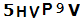Our Job Portal : www.JobListIndia.com
Students Hotline : 93807 61234

# Fibonacci Sequence

23
Mar
3/23/2021 10:11 PM

Fibonacci Sequence

Leonardo of Pisa (Fibonacci) is one of the greatest mathematicians of the Middle Ages. Before the formulation of Fibonacci numbers, Ancient Greeks and Indians used the similar sequence in many areas of science and arts. Therefore, despite the large use of Fibonacci numbers in physics and mathematics, it also could be found in nature and architecture. Fibonacci numbers is a linear sequence of natural numbers, where each subsequent number is the sum of the previous two. The numerical series that today bears Fibonacci name grew out of the problem with rabbits that mathematician described in his book Liber Abaci. Despite the fact that the sequence is called in honor of Fibonacci, the real person who defined it was Leonardo of Pisa. At the request of his father, who wanted Leonardo to become a good trader, he moved to Algeria and studied the art of calculation. Leonardo of Pisa never called himself Fibonacci. Fibonacci sequence could be found in nature, biology, and science. Due to the Fibonacci discovery, the phenomena called phyllotaxis (leaf arrangement) emerged. Phyllotaxis is the rule according to which, the seeds of a sunflower are arranged in two rows of spirals.: one row goes clockwise and the other against the clock. Moreover, the DNA consists of vertical spirals whose length and width corresponds to 34 and 21 respectively. The sequence of that numbers comes from Fibonacci sequence. The lengths of the phalanges of the person’s fingers are roughly the same as the Fibonacci numbers. The sleeves of many spiral galaxies are arranged in accordance with this sequence. An excellent example is a galaxy where Earth is situated. To conclude, Fibonacci numbers is a linear sequence of natural numbers, where each subsequent number is the sum of the previous two. Fibonacci was the pseudonym of Leonardo of Pisa who presented the famous sequence by providing the mathematical task about rabbit population. Fibonacci sequence could be found in nature and many areas of science.

The article was composed and written by professional writer Olivia Adamson, more her papers you can find at freshessay writing service.Tags : education history
Categories : uncategorized
 Name: * E-Mail: * Website: Comment: * Notify me of follow-up comments via email. Security Code: *